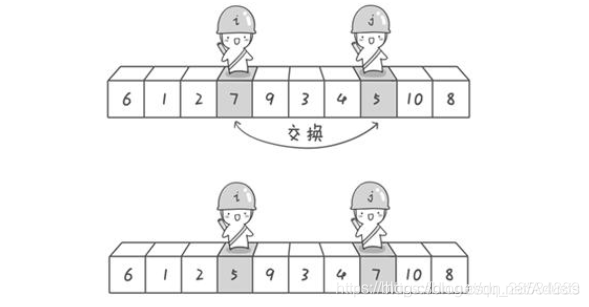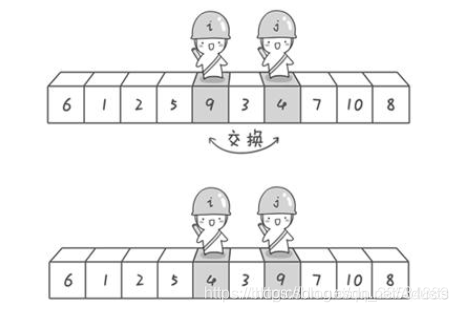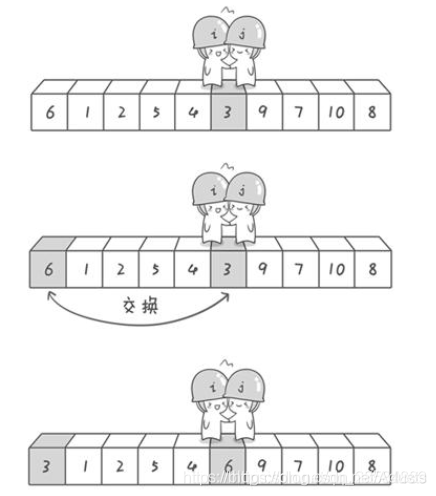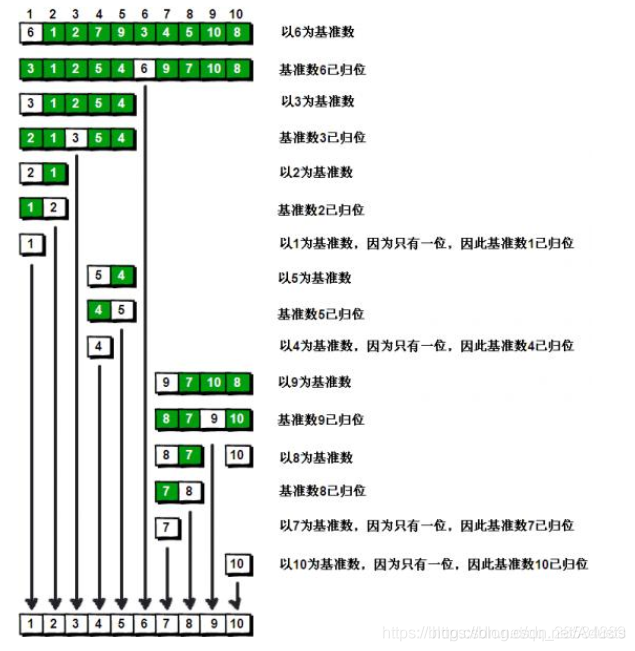Python 快速排序法（转）二、首先 哨兵j 开始出动。2  1  3  5  4
OK，现在3已经归位。

1  2  3 4  5  6 9  7  10  8

1  2  3 4  5  6  7  8 9  10#快速排序法：

def quickSort(arr,left,right):#arr:待排序的数列；left:数列开始的下标索引；right:数列结束的下标索引

if left > right:#如果开始索引大于结束索引
return

temp=arr[left];#取最左边的数为 基准数
i,j=left,right

while i!= j:#现从右边开始找
while arr[j]>=temp and i<j:#当前数值大于基准数
j-=1

while arr[i]<=temp and i<j:#当前数值小于基准数
i+=1

if i<j:#交换两个数再数组中的位置
t=arr[i]
arr[i]=arr[j]
arr[j]=t

#将 基准数 归位
arr[left]=arr[i]
arr[i]=temp

quickSort(arr,left,i-1)#递归：继续处理左边的
quickSort(arr,i+1,right)#递归：继续处理右边的

#测试
arr=[10,7,8,8,9,1,5]
n=len(arr)
quickSort(arr,0,n-1)
print(“排序后的数组：”)
for i in range(n):
print(“%d” %arr[i])